```<?php

require_once (dirname(__FILE__) . "/config.inc.php");
require_once (dirname(__FILE__) . "/Element.php");
require_once (dirname(__FILE__) . "/fetch.php");

// Check if char is a whitespace character.
function char_is_white_space(\$char)
{
if (\$char == " " || \$char == "\t" || \$char == "\n")
{
return True;
}
return False;
}

// Move the file pointer along until it finds a non-whitespace character.  If
// the user wants to the next non-whitespace character, first move the file
// pointer one step.
function skip_white_space(\$fp, &\$char, \$next=False)
{
if (\$next == True || char_is_white_space(\$char) == True)
{
while (char_is_white_space(\$char = fgetc(\$fp)) == True) {}
}
}

// Move the file pointer along until it finds the desired character.  If the
// user wants the next instance of the character, first move the pointer one
// step away from its current position.
function move_to_char(\$fp, &\$char, \$sought, \$next=False)
{
if (\$next == True || \$char != \$sought)
{
while ((\$char = fgetc(\$fp)) != \$sought && !feof(\$fp)) {}
}
}

// Skip any initial white space.  Read and store characters until white space or
// a bracket is reached.
function parse_name_or_id(\$fp, &\$char, &\$data=NULL)
{
skip_white_space(\$fp, \$char);
while (
!char_is_white_space(\$char) && \$char != OPEN_ELEMENT_CHAR &&
\$char != CLOSE_ELEMENT_CHAR && !feof(\$fp))
{
\$data .= \$char;
\$char = fgetc(\$fp);
}
}

// Create a new element & store it as the first child of the current element or
// the next child of a parent element.
function build_new_element(\$fp, &\$char, &\$element, \$ii)
{
\$new = new Element();
if (\$ii == 0)
{
\$element->data = \$new;
}
else
{
\$element->next = \$new;
}
parse_element(\$fp, \$new, \$char);
\$element = \$new;
}

// Read the last component of an element.  If there is a bracket in the data,
// treat it as a new element, and add its contents to the current element.  If
// not, treat the data as a string, and store it.
function parse_data(\$fp, &\$char, \$element)
{
skip_white_space(\$fp, \$char);
while (\$char != CLOSE_ELEMENT_CHAR && !feof(\$fp))
{
for (\$ii = 0; \$char == OPEN_ELEMENT_CHAR; \$ii++)
{
build_new_element(\$fp, \$char, \$element, \$ii);
\$char = fgetc(\$fp);
skip_white_space(\$fp, \$char);
\$flag = True;
}

if (\$flag == True) break;

\$element->data .= \$char;
\$char = fgetc(\$fp);
}
}

// Decide which component is being parsed and dispatch to the appropriate
// parsing function.
function parse_component(\$fp, \$element, &\$char, \$component)
{
switch (\$component)
{
case NAME_COMPONENT:
parse_name_or_id(\$fp, &\$char, \$element->name);
break;
case ID_COMPONENT:
parse_name_or_id(\$fp, &\$char, \$element->id);
break;
case DATA_COMPONENT:
parse_data(\$fp, &\$char, \$element);
break;
}
}

// Ensure we've found an element by moving to the first open bracket.  Parse the
// contents within the brackets (one or more elements).
function parse_element(\$fp, \$element, &\$char=NULL)
{
move_to_char(\$fp, \$char, OPEN_ELEMENT_CHAR);
\$char = fgetc(\$fp);
for (\$ii = 0; \$ii < COMPONENT_COUNT; \$ii++)
{
parse_component(\$fp, \$element, \$char, \$ii);
}
}

// Parse a file or subset of a file, creating a tree of elements.
function run_parse(\$file_name, \$id=NULL)
{
if (!file_exists(\$file_name)) return;

\$elements = fetch_elements(\$file_name, \$id);
\$elements = (\$id) ? array(\$elements) : \$elements;
foreach (\$elements as \$element)
{
\$message .= \$element->convert_to_tabbed_string() . "\n";
\$count += \$element->count();
\$depth = max(\$element->measure_depth(), \$depth);
}
\$message .= "DEPTH: \$depth\n";
\$message .= "TOTAL: \$count\n";

return \$message;
}
```
```<?php

require_once (dirname(__FILE__) . "/Element.php");
require_once (dirname(__FILE__) . "/parse.php");

// Parse the name of an element without storing it.
function skip_name(\$fp, &\$char)
{
parse_name_or_id(\$fp, \$char);
}

// Read the id of the element listed at the current position.
{
skip_name(\$fp, \$char);
parse_name_or_id(\$fp, \$char, \$id);

return \$id;
}

// Move file pointer to element with requested ID.  After finding the ID, move
// the file pointer backward past the element name and open bracket.
function move_to_element_by_id(\$fp, &\$char=NULL, \$id)
{
while (!feof(\$fp))
{
move_to_char(\$fp, \$char, OPEN_ELEMENT_CHAR);
\$position = ftell(\$fp);
\$saved_char = \$char;
\$char = fgetc(\$fp);
if (read_element_id(\$fp, \$char) == \$id) break;
}

if (feof(\$fp)) return;

fseek(\$fp, \$position);
\$char = \$saved_char;
}

// Find and return the element identified by the requested ID.
function fetch_element_by_id(\$file_name, \$id)
{
\$fp = fopen(\$file_name, 'r');
move_to_element_by_id(\$fp, \$char, \$id);
\$element = new Element();
parse_element(\$fp, \$element, \$char);

return \$element;
}

// Return an array of all elements in a file.
function fetch_elements_by_file(\$file_name)
{
\$fp = fopen(\$file_name, 'r');

skip_white_space(\$fp, \$char, True);
while (!feof(\$fp))
{
\$elements[] = new Element();
parse_element(\$fp, \$elements[count(\$elements)-1], \$char);
\$char = fgetc(\$fp);
skip_white_space(\$fp, \$char);
}

return \$elements;
}

// Dispatch to appropriate function depending upon whether single or
// multiple elements are being requested.
function fetch_elements(\$file_name, \$id=NULL)
{
if (file_exists(\$file_name))
{
if (\$id == NULL)
{
\$elements = fetch_elements_by_file(\$file_name);
}
else
{
\$elements = fetch_element_by_id(\$file_name, \$id);
}
}

return \$elements;
}
```
18.207.252.123
18.207.252.123
18.207.252.123

June 5, 2016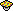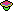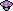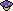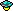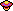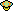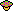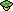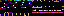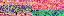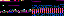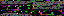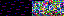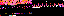↠ RSS Feed ↞